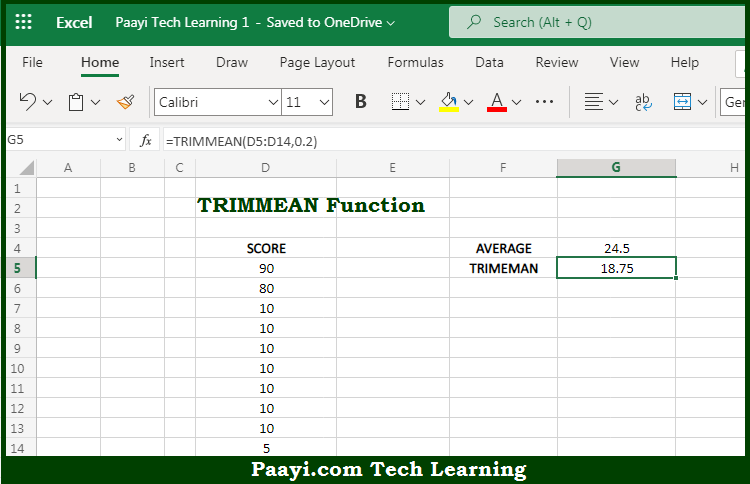# Learn How to Use Microsoft Excel TRIMMEAN Function

Written by | 0 Comments | 581 Views

In this article, you will learn how to use the Microsoft Excel TRIMMEAN function and its prime function in Microsoft Excel. You will also get to know the Microsoft Excel TRIMMEAN function return value and syntax with the help of some examples.

Microsoft Excel TRIMMEAN Function

The main purpose of the Microsoft Excel TRIMMEAN function is to calculate the mean excluding outliers. That implies, with the help of the TRIMMEAN function you can able to calculate the mean or average while excluding outliers. It should be noted that the number of data points to exclude is provided as a percentage. So, with the help of the TRIMMEAN function, you can able to calculate the mean excluding outliers.

Return Value of TRIMMEAN Function

The return value will be the calculated mean.

Syntax of TRIMMEAN Function

=TRIMMEAN(array, percent)

Where the arguments:

• array: These are the values to trim and average.
• percent: This is the number of data points to exclude from the calculation.

## How to Use Microsoft Excel TRIMMEAN Function?So we know that Microsoft Excel TRIMMEAN function you can able to calculate the mean excluding outliers. That implies, with the help of the TRIMMEAN function you can able to calculate the mean or average while excluding outliers. It should be noted that the number of data points to exclude is provided as a percentage. So, with the help of the TRIMMEAN function, you can able to calculate the mean excluding outliers.